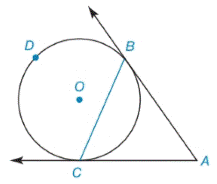Chapter 6.2, Problem 9EElementary Geometry For College St...

7th Edition
Alexander + 2 others
ISBN: 9781337614085

Solutions

Chapter
SectionElementary Geometry For College St...

7th Edition
Alexander + 2 others
ISBN: 9781337614085
Textbook Problem

Given: Tangent A B →  and A C → to ⊙ O m ∠ A C B = 68 ° Find: a) m B C ⌢ b) m B D C ⌢ c) m ∠ A B C d) m ∠ ATo determine

(a)

To find:

mBC when AB and AC are tangent to O, mACB=68°.

Explanation

Corollary:

The measure of an angle formed by a tangent and a chord drawn to the point of tangency is one-half the measure of the two intercepted arc.

Calculation:

mBC when AB and AC are tangent to O, mACB=68°.

To determine

(b)

To find:

mBDC when AB and AC are tangent to O, mACB=68°.

To determine

(c)

To find:

mABC when AB and AC are tangent to O, mBC=136°.

To determine

(d)

To find:

mA when AB and AC are tangent to O, mBC=136° and mBDC=224°.

Still sussing out bartleby?

Check out a sample textbook solution.

See a sample solution

The Solution to Your Study Problems

Bartleby provides explanations to thousands of textbook problems written by our experts, many with advanced degrees!

Get Started

Solve the equations in problem 1-6. 2.

Mathematical Applications for the Management, Life, and Social Sciences

In Exercises 4562, find the values of x that satisfy the inequality (inequalities). 51. x + 1 4 or x + 2 1

Applied Calculus for the Managerial, Life, and Social Sciences: A Brief Approach

Polynomial Inequalities Solve the inequality. 94. x3 3x2 4x + 12 0

Precalculus: Mathematics for Calculus (Standalone Book)

Evaluate the integral. 19. z3ezdz

Single Variable Calculus: Early Transcendentals

Multiply. (2cos+3)(4cos5)

Trigonometry (MindTap Course List)

True or False: If converges absolutely, then it converges conditionally.

Study Guide for Stewart's Single Variable Calculus: Early Transcendentals, 8th

True or False: If converges and converges, then converges.

Study Guide for Stewart's Multivariable Calculus, 8th

In Exercises 116, determine whether the argument is valid. pqqrpr

Finite Mathematics for the Managerial, Life, and Social Sciences